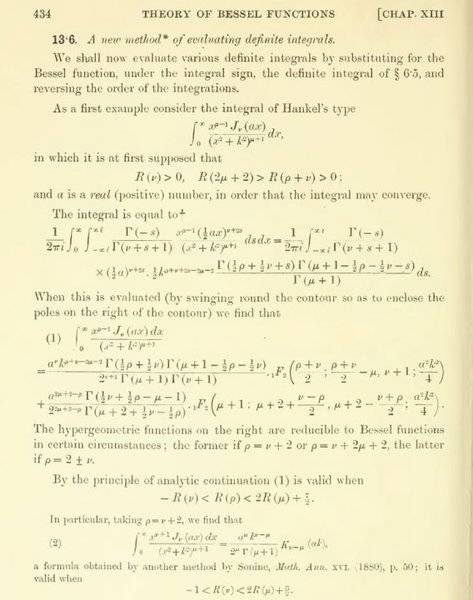# Integrals of the Bessel functions of the first kind

Hi Physics Forums.

I am wondering if I can be so lucky that any of you would know, if these two functions -- defined by the bellow integrals -- have a "name"/are well known. I have sporadically sought through the entire Abramowitz and Stegun without any luck.

$f(x,a) = \int_0^\infty\frac{t\cdot J_0(at)}{t^2 + x^2}\,\mathrm{d}t$

and

$g(x,a) = \int_0^\infty\frac{t^2\cdot J_0(at)}{t^2 + x^2}\,\mathrm{d}t$

where $J_0(x)$ is the Bessel function of the first kind.

fzero
Homework Helper
Gold Member
$f(x,a) = \int_0^\infty\frac{t\cdot J_0(at)}{t^2 + x^2}\,\mathrm{d}t$

This one is 6.532.4 in Gradshteyn and Ryzhik, equal to ##K_0(ax)## for ##a>0, \text{Re}~x>0##.

$g(x,a) = \int_0^\infty\frac{t^2\cdot J_0(at)}{t^2 + x^2}\,\mathrm{d}t$

I think this one falls into a class of integrals solved in Watson's A Treatise on the Theory of Bessel Functions, expressible as hypergeometric functions. I attached the relevant page: